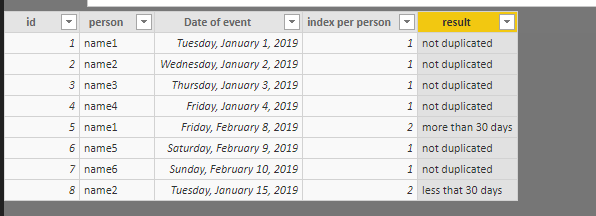cancel
Showing results for
Did you mean:Anonymous
Not applicable

## Help! Is the Name repeated? then if calculate a date difference if so

Column1: id

Column2: person

Column3: Date of event

This is my table above, i want to identify if the person is duplicated in the table if so, is the date difference less that 30 days.

i have a few ways to approach this but i cannot seem to get something to work.

Any help is appreciated.

Thanks

1 ACCEPTED SOLUTIONCommunity Support

Hi @Anonymous ,

One sample for your reference, here we can create two calculated columns as below.

```index per person =
RANKX (
FILTER ( Table1, Table1[person] = EARLIER ( Table1[person] ) ),
'Table1'[Date of event],
,
ASC,
DENSE
)
```
```result =
VAR index1 = Table1[index per person] - 1
VAR date1 =
CALCULATE (
MAX ( 'Table1'[Date of event] ),
FILTER (
Table1,
Table1[person] = EARLIER ( Table1[person] )
&& Table1[index per person] = index1
)
)
VAR difference =
DATEDIFF ( date1, 'Table1'[Date of event], DAY )
RETURN
IF (
ISBLANK ( difference ),
"not duplicated",
IF ( difference > 30, "more than 30 days", "less that 30 days" )
)
```Community Support Team _ Frank
If this post helps, then please consider Accept it as the solution to help the others find it more quickly.Community Support

Hi @Anonymous ,

One sample for your reference, here we can create two calculated columns as below.

```index per person =
RANKX (
FILTER ( Table1, Table1[person] = EARLIER ( Table1[person] ) ),
'Table1'[Date of event],
,
ASC,
DENSE
)
```
```result =
VAR index1 = Table1[index per person] - 1
VAR date1 =
CALCULATE (
MAX ( 'Table1'[Date of event] ),
FILTER (
Table1,
Table1[person] = EARLIER ( Table1[person] )
&& Table1[index per person] = index1
)
)
VAR difference =
DATEDIFF ( date1, 'Table1'[Date of event], DAY )
RETURN
IF (
ISBLANK ( difference ),
"not duplicated",
IF ( difference > 30, "more than 30 days", "less that 30 days" )
)
```Community Support Team _ Frank
If this post helps, then please consider Accept it as the solution to help the others find it more quickly.